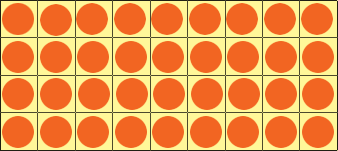My third grader brought home this math problem: Here are five arrays for 36. 1. Lable the dimension for each array. 2. Write a multiplicatiuon sentence for each arrray. Hi, Here is one array of 36 items.Its dimensions are 4 and 9, or you might say this is a 4 by 9 array. We always mention the rows first and then the columns. The multiplication sentence is 49 = 36 Penny